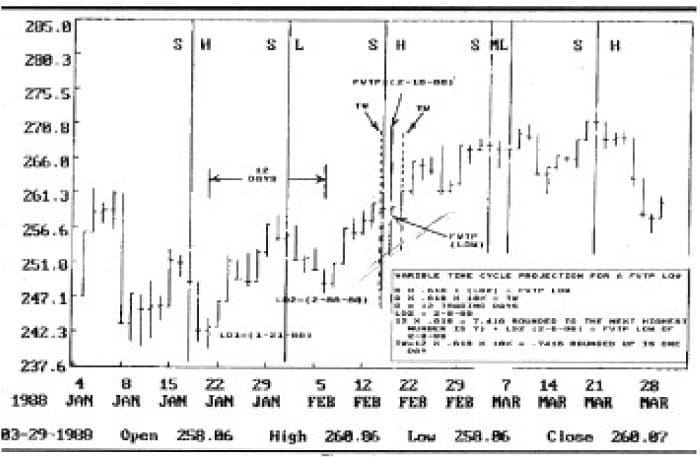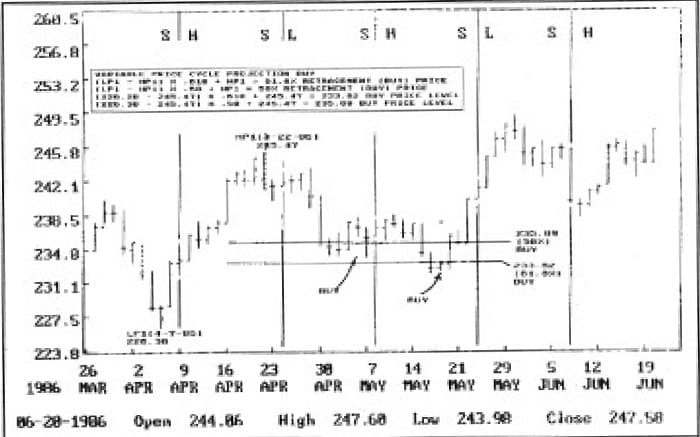Astro Blogs

# Cycles: Predicting Price & Time By Jeff Rickerson

Rate this post

In the never ending quest to predict C the never ending quest to the futures markets (or the future for that matter) I have found that price/time series analysis; or the study of rhythmic behavior (both in nature and financial markets) is the key to unlimited profit potential in futures trading. My interest in cycles was born out of my interest in commodity futures. Recurring cycles in commodities as well as in other areas have always fascinated me. I was curious as to why they recured with such predictive regularity. It was my intense curiosity and interest in why things in nature (or for that matter, the entire universe) occur and recur in regular time intervals with such predictability.My research has isolated several major cycles which recur with startling precision. This precision, which can be precisely projected, increased my curiosity as to why this occurs; both in nature and ﬁnancial markets.

#### Read Book: Turning Point Analysis In Price & Time

The theory of cycle analysis is that certain events will occur within the cycle triggering prices (or other naturally occurring events) to change and move in the expected direction and duration of the cycle during a speciﬁc time interval. Before we begin discussing cycles we ﬁrst must have an understanding of cycles. The word cycle comes from the Greek word circle. Simply stated, a cycle is the coming around to the point of beginning.Figure 1.

However, we want to be more speciﬁc in our measurement of recurring cycles in futures. We want to be precise as to when the cycle will begin and end. In cycles we are looking for rhythm. Rhythm is another Greek derivative which means “Measurable Time”. A cycle with rhythm is one that comes around to the point of beginning at regular and measurable time intervals. A rhythmic cycle has a tendency of being periodic, or one of periodicity. This is to say that the cycle repeats itself in a regular pattern of wavelengths at regular and precise time intervals. This is similar to the now famous Elliott Wave theories. A wavelength is the length of a wave (high to high or low to low of a price wave or other wave, etc., that is being measured). An oscillation is a single wave in a series of waves. An oscillation is a ﬂuctuation between a variable or ﬁxed time intervals. There is one last deﬁnition that is needed for your understanding of cycles. Frequency is the number of waves/oscillations in a speciﬁc time interval. This is import’ant because there are several short term waves in one long term wave. This creates both short term and long term cycles.

Where does all the above ﬁt into our analysis and trading the stock market or other commodity markets? Well, ﬁrst of all many economists, market analysis, ﬁnancial consultants and investors/traders believe that all markets follow the “random walk” theory. This is to say that all markets are random and follow no pattern at all; that the markets just exist and that price/time movement is created from randomness and that history does not repeat itself in predictable intervals.

#### Read Book: A Random Walk Down Wall Street

If the random walk theory is true then why do markets seem to mysteriously and suddenly snap back (after having been distorted) into speciﬁc cycles of rhythmic patterns that can be precisely determined before they occur. The speciﬁc cycles of periodicity I am referring to can be measured and have been proven. It is this fact that markets (or other naturally occurring phenomenon) do have a speciﬁc and regular degree of predictability that I believe discredits the random walk theory of the markets as a 100 percent reason for why the markets behave as they do. I believe that the random walk theory is only 10 percent of the equation and occurs during time periods of distortion caused by such events as positive/negative government reports, threats of war or natural disasters, etc. However, even these events can be accurately calculated using the same cycle analylsis so the entire theory of random walk can easily be discredited.

In order to proﬁt from cycle analysis in trading stock and commodity markets you must ﬁrst isolate the minor and major cycles in those markets. You must then analyze those cycles for statistical signiﬁcance on many years of historical data. Next those cycles must be able to be projected into the future and occur on or within a speciﬁc time period in order to be statistically signiﬁcant — that is not just by chance. In my own cycle analysis I have used both ﬁxed and variable calculations for projecting both price and time. A variable cycle projection is based on actual past high and low price/time points which change as new highs/lows occur. A fixed cycle projection is based upon a predetermined and set price/time equation that does not change regardless of past, present, or future price/time and continues into inﬁnity.

In this article we will be concerned with only one variable calculation. I have chosen variable cycle projections for three reasons: (1) simplicity, (2) it works on all markets, (3) I am able to give a simple example that will be useful in current trading. Fixed price/time cycle projections involve my other proprietory knowledge that I have programed into a computer along with other variable cycle projections. Combining the two makes for a most proﬁtable trading system. In making a variable cycle calculation for price and time please refer to Figures 1-4. In reference to Figures 1-4 the following equations and abbreviations will be used.Figure 2.

First pick two low points (L1 & L2) [See Figure 1]. Count the distance (trading days) between the two low points. Multiply this distance by the Fibonacci number .618 (round up to the next highest number). This gives you the number of days that you add to the most recent low (L1) that has occured which you chose. The resulting answer is the FvTP day which the next low will occur in the variable time sequence. Take the number of days which you added to low point number one (L1) and multiply that by 10 percent. This gives you a time window or the number of days on either side of the FvTP date that prices could also reverse. You would do the same for two high points (H1 & H2) [See Figure 2] in order to calculate the next FvTP high time point.Figure 3.

The above is one simple way to calculate a variable time cycle projection. In order to calcuate a variable price cycle projection use the variable price cycle equation. From a major low or high point calculate a 61.8 percent and-a 50 percent price retracement. This will give you a price window that prices have a high probability of reversing. Refer to Figure 3 and Figure 4 and plug in the appropriate values in the equation.Figure 4.

My current research and newest discoveries has allowed me to expand the basic deﬁnition of cycles; why they occur, and why they occur when they do using both variable and ﬁxed price/time equations. I do not have enough room in one article to reveal the sequence of events that lead up to my discovery or even to reveal the discovery. However, in this article I hope I have introduced you to an important aspect of cycle analysis.